## Section48.6Telescope

Although accounts vary as to who invented the first telescope, but records suggest that Galileo Galelei built his first telescope using two converging lenses in a tube. The lens towards the object is called the objective lens and the one close to the eye is called the eyepiece or ocular. Galileo was the first one to make observations in astronomy using a telescope.

Galileo's telescope uses refraction by objective lens to focus light from distance objects. We call these types of telescopes refracting telescopes. But, images in these types of telescopes suffer from blurring due to chromatic aberration, in which a rainbow of colors is seen over and around the image. This happens because of different refractive indices of differently colored light, resulting into different amount of bending.

To solve the chromatic aberration problem, Isaac Newton replaced the objective lens by a concave mirror. The telescopes that do that are called reflecting telescopes. There are several designs for reflecting telescopes in use today. The mirror here is called objective or objective mirror.

The magnifying power $M_\text{telescope}$ of either type of telescope is given by the ratio of the focal lengths of the objective and the eyepiece, viz., $f_\text{o}$ and $f_\text{e}\text{.}$ The absolute value of $M$ is

\begin{equation*} |M_\text{telescope}| = \frac{f_\text{o}}{f_\text{e}}. \end{equation*}

The light gathering ability and speed of a telescope is given by its F/#, which is the ratio of the focal length of the objective to the aperture diameter of the opening through which light enters the telescope.

\begin{equation*} F/\# = \frac{f_\text{o}}{D}. \end{equation*}

Most of the research telescopes are now of reflecting type. One of the largest telescopes of this kind is the Hale 200-inch or 5-meter built on Mount Palomar in southern California (Visit the Hale Observatory website). The measurement of 200 inches here refers to the diameter of the opening of the mirror, giving it light collecting area of about $218.2\text{ sq.ft}\text{.}$ The mirror has focal length of 660 inches, giving the 200-inch Hale an F/# of 3.3. The building of Hale at Palomer was a great engineering achievement.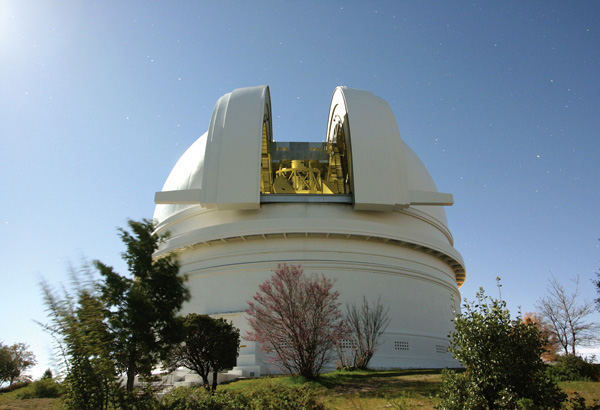Figure 48.6.1. The 200-inch Hale Telescope at Palomar Observatory in San Diego County, California, US. Credits: Palomer/Caltech.

In 2012, the largest telescope was the 10 meter Keck telescope at the Keck observatory on the summit of the dormant Mauna Kea volcano, in Hawaii (Visit the Keck Observatory website). At the Keck observatory there are two 10 meter telescopes. They are not single mirror, but instead made up of 36 hexagonal mirrors each. Furthermore, the two telescopes on the Keck can work together through an interference which increases their power to an effective 85-meter aperture diameter! This gives the astonishing collection area of $5,675\text{ m}^2$ or $61,100\text{ sq.ft}\text{.}$

### Subsection48.6.1Refracting Telescope

Historically, refracting telescope was the first telescope to be used for observing astronomical objects. Although the accounts vary as to who invented the first refracting telescope, but records suggest that Galileo Galelei built his own refracting telescope and was the first one to make observations in astronomy using a telescope.

Refracting telescope is similar to a microscpe. It also uses two lenses - the first of these lenses facing the object is called the objective or objective lens, and the second lens near the eye is called the eyepiece. While in microscope, both lenses were used to magnify, the task of the objective in a telescope is to bring the far away object near and then eyepiece is used to magnify this real image.

Figure 48.6.2 shows the optical setup of a telescope. The objective forms a real image $\text{Q}_1$ of a star that lies in the direction of $\theta_\text{o}\text{.}$ This image forms in the focal plane of the second focal plane of the objective lens. The first focal plane of the eyepiece is very close to it and left of it sso that when we use the real image as the object for the eyepiece, we get a virtual image in the direction $\theta_\text{i}\text{.}$

The magnification or power of a telescope is given by the ratio of the angle subtended by the image to that subtended by the object.

\begin{equation*} M_\text{telescope} = \frac{\theta_i}{\theta_o}. \end{equation*}

We can identify a triangle between the two lenses using the chief rays to the image $\text{Q}_1$ from the objective and the ray in the direction of the final virtual image as shown in Figure 48.6.3. Then, angles $\theta_\text{o}$ and $\theta_\text{i}$ can be related to the focal lengths of the two lenses since their separation is very close to the sum of the foci.

\begin{equation*} \text{separation of lenses } = f_\text{o} + f_\text{e}. \end{equation*}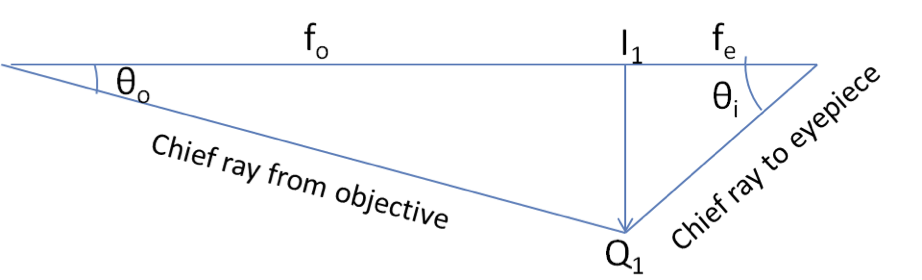Figure 48.6.3. Geometry of the chief rays with the assumption that the distances between the image $\text{I}_1\text{Q}_1$ and the lenses are each approximately equal to the respective focal lengths.

By using tthe geometry in Figure 48.6.3 and assuming small angles, we can rewrite the magnification of a refractive telescope as

\begin{align*} M_\text{telescope} \amp = \frac{\theta_\text{i}}{\theta_\text{o}}\approx = \frac{\tan\theta_\text{i}}{\tan\theta_\text{o}}\\ \amp = -\frac{\text{I}_1\text{Q}_1}{f_\text{e}} \times \frac{f_\text{o}} {\text{I}_1\text{Q}_1} = - \frac{f_\text{o}}{f_\text{e}}, \end{align*}

where I have introduced a minus sign to take care of the negative direction of the direction to the final image. Thus, magnifying power of a telescope simply depends on the foci of the objective and the eyepiece. Since power of a lens is inverse of their focal length, we can also write the magnification as

\begin{equation*} M_\text{telescope} = - \frac{f_\text{o}}{f_\text{e}} = - \frac{P_\text{e}}{P_\text{o}}. \end{equation*}

Therefore, higher power eyepiece and lower power objective will give you more magnification.

Typical eyepieces have focal lengths of $2.5\text{ cm}\text{,}$ and $1.25\text{ cm}\text{.}$ If the objective of the telescope has a focal length of $1\text{ meter}\text{,}$ then these eyepieces would result in magnifications of 40-times, and 80-times respectively. The angular magnifications would make the image appear 40-times, or 80-times closer.

In a refracting telescope objective lens collects light from the source. For fainter objects in the sky you need large diameter objective lens. The largest refracting telescope in the world is the 40 inch diameter Yerkes telescope operated by the University of Chicago and located at Lake Geneva, Wisconsin (Figure 48.6.4).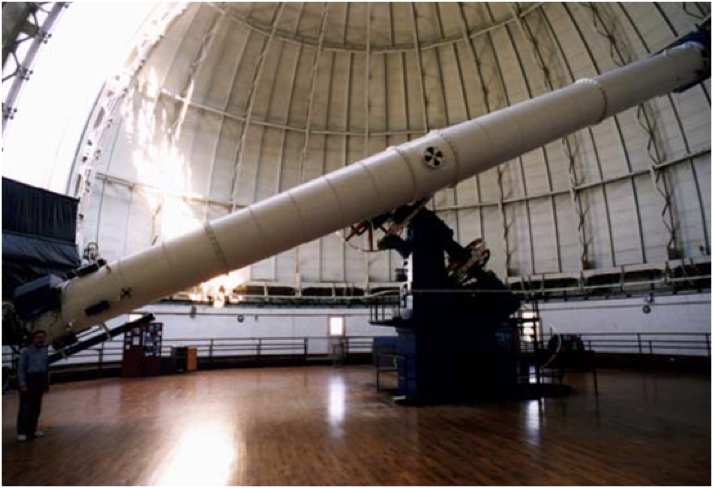Figure 48.6.4. Yerkes observatory in Wisconsin (USA) built in 1897 has a large objective lens of $40\text{ inches}$ in diameter and tube length of $62\text{ feet}\text{.}$ (Photo credit: Yerkes Observatory, University of Chicago)

It is very difficult and expensive to build large refracting telescopes. You need large defect free lenses, which in itself is a technically demanding task. Refracting telescope basically looks like a tube with support structure to rotate it in different directions.

A refracting telescope suffers from a number of defects. The aberration of lenses causes the image to be blurred. Also as the lenses become thicker for larger lenses more light is absorbed making the faint stars more difficult to observe. Large lenses are also very heavy and deform under their own weight. Some of these problems with refracting telescope is addressed by avoiding refraction for collecting light. Instead a curved mirror is used for the objective. Such telescopes were first designed by Isaac Newton. These telescopes are called the reflecting telescopes which we discuss next.

### Subsection48.6.2Reflecting Telescope

In the reflecting telescope, also known as a reflector, light rays from a distant source fall upon the surface of a concave mirror fixed at the bottom end of the tube. The concave mirror focuses the rays on its focal plane. To observe the focused image Newton used a design in which the focused light from the concave mirror was reflected to one side of the tube into an eyepiece as shown in Figure 48.6.5. This arrangement is common in many amateur telescopes and is called Newtonian design.

Some telescopes reflect the light back towards the middle of the concave mirror by using a convex mirror as shwon in Figure 48.6.6. In this arrangement the light-gathering concave mirror has a hole in the middle through which light emerges to be incident on the eyepiece lens. This arrangement of the objective and eyepiece is called Cassegrainian design.\ Most big telescopes, including Hubble telescope (see Figure 48.6.7), are of this design. There are other arrangements also possible. Sometimes one puts a light detector right at the spot where light is focused by the curved mirror.

Hubble telescope shown in Figure 48.6.7, put in the orbit around earth in 1990, is another large reflecting telescope with the primary mirror of diameter 2.4 meter. That makes $f_\text{o}= 2.4/4 = 0.6\text{m}\text{.}$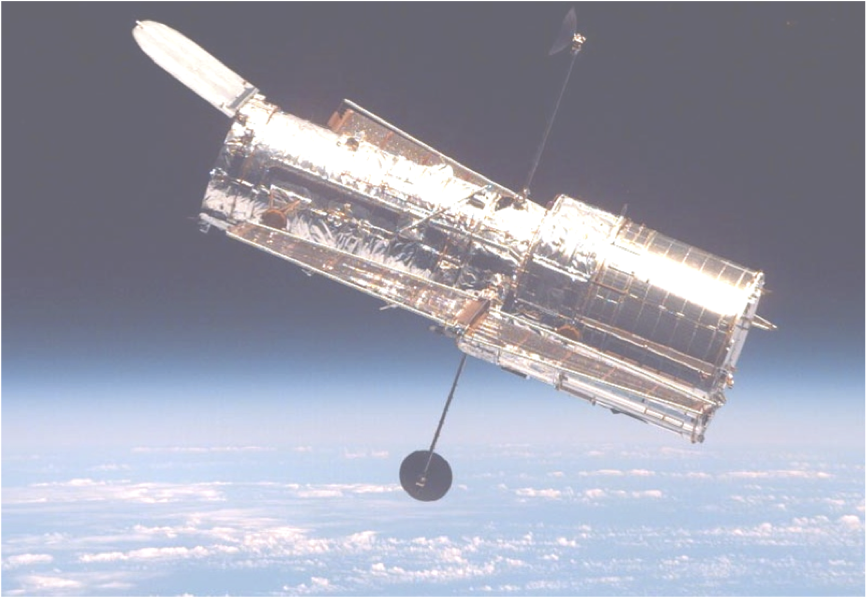Figure 48.6.7. Hubble Space Telescope as seen from the Space Shuttle Discovery on mission STS-82. (NASA, Feb 1997, see http://hubble.nasa.gov)

The formula for the angular magnification $M$ of a reflecting telescope is same as that of the refracting telescope.

\begin{equation} M_\text{telesccope} = - \frac{f_\text{o}}{f_\text{e}} = - \frac{R_\text{o}}{2f_\text{e}},\tag{48.6.1} \end{equation}

where $f_\text{o}$ is half of the radius of curvature, $R_\text{o}\text{,}$ of the objective mirror and $f_\text{e}$ is the focal length of the eyepiece. You can see that making large objective not only helps out with collecting more light into the telescope it also helps with the magnification of the image.

### Subsection48.6.3Resolving Power of a Telescope

An important use of a telescope is to be able to resolve images of two nearby stars. The image seen through a telescope is affected by a number of factors, such as poor optics and blurring by atmosphere. When a telescope, such as Hubble, is placed above the atmosphere and optical aberrations discussed below are eliminated, resolving two point-like objects is still limited by diffraction effects, which cannot be eliminated since it is fundamental aspect of wave nature of light.

We will discuss diffraction in a later chapter. Here, we summarize some of the important features of resolution of two stars in approximately same direction. Suppose we project the image of a single star on a CCD detector. What we will observe is that there is a central bright disk in the direction of the star, called the Airy disk, surrounded by dark ring which is alternately surrounded by less and less bright and dark rings. This is due to the diffraction effect.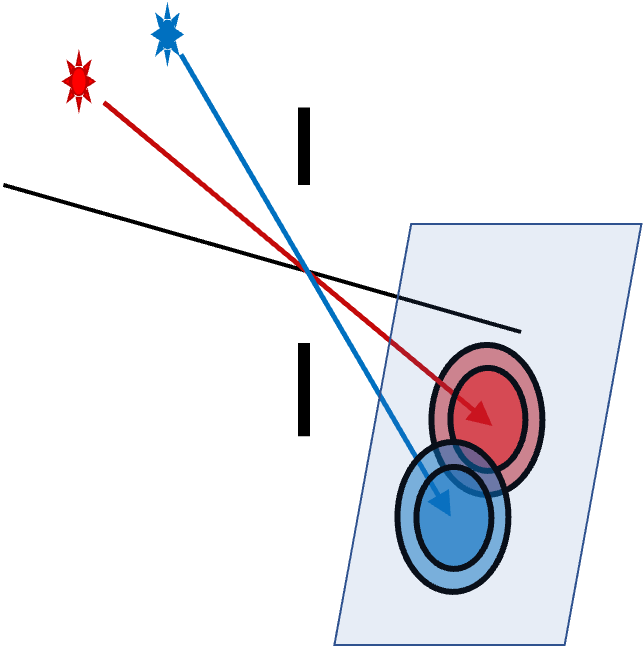Figure 48.6.8. Diffraction pattern on the CCD detector from two stars.

Quantitatively, sizes of the rings depend upon the objective's aperture $D$ and the wavelength $\lambda$ of light. From the center of the aperture, which will be at the center of the lens of the CCD camera if telescope is equipped with a CCD detector, the central bright disk will be spread over an angle $\Delta\theta$ given by

\begin{equation*} \Delta\theta = \frac{1.22\lambda}{D}. \end{equation*}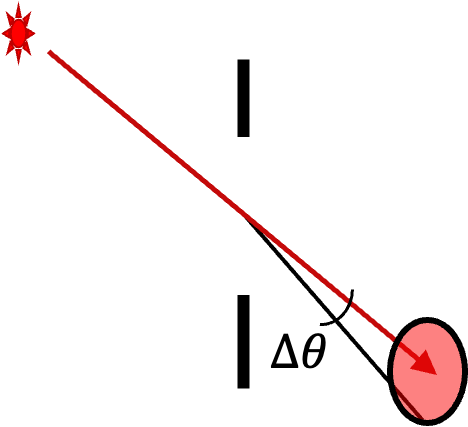Figure 48.6.9. Angle $\Delta\theta\text{.}$

Each star will form its blob of this size on the screen. If the angular separation of the two stars was less thin this angle, it will difficult to tell whther you have two stars or one - they are said to be not resolved. This is called the Raleigh criterion. This is a rule of thumb that works well. We call this Raleigh limit of resolution of the telescope and loosely speak of this as a limit of resolution.

Although Raleigh criterion provides a useful metric of resolution of images of two nearby stars, one can often go beyond it. There is another criteria, called Sparrow criterion, which looks at the presence of any dip in the image and use that dip to separate the images of the two stars. We will not go into that here.

A telescope has an objective of focal length equal to $1100\text{ mm}\text{.}$ When you use an eyepiece that has focal length $20\text{ mm}\text{,}$ what will be the magnification?

Hint

It is the ratio of foci.

$55\text{.}$

Solution

Answer is obtained simply by taking the ratio of the foci. We ignore the sign here.

\begin{equation*} |M| = \frac{f_\text{o}}{f_\text{e}} = \frac{1100}{20} = 55. \end{equation*}

The first telescope that Galileo is said to have made had two lenses in a tube with objective of focal length $98\text{ cm}$ and the eyepiece had the focal length of $5\text{ cm}\text{.}$ What was its magnification?

Hint

It is the ratio of foci.

$55\text{.}$

Solution

Answer is obtained simply by taking the ratio of the foci. We ignore the sign here.

\begin{equation*} |M| = \frac{f_\text{o}}{f_\text{e}} = \frac{98\text{ cm}}{5\text{cm}} = 19.6. \end{equation*}

(a) A reflecting telescope has a concave mirror with opening diameter of $24\text{ inches}$ and focal length $10\text{ feet}\text{.}$ What is the F/# of the telescope?

(b) The image in the reflector in (a) is to be viewed through a number of eyepieces of focal lengths $4\text{ mm}\text{,}$ $10\text{ mm}\text{,}$ $25\text{ mm}\text{,}$ and $35\text{ mm}$ are avilable. Find the magnifications in each case.

Hint

(a) Use ratio of focal lengths in the same units. (b) Make sure you have the same units. Once you find $M$ for one, you can get the others by simply scaling.

(a) $5\text{,}$ (b) $762,\ 304.8,\ 121.9,\ 87.1\text{.}$

Solution 1 (a)

(a) The F/# is obtained from the ratio of focal length and the diameter of the aperture.

\begin{equation*} F/\# = \frac{f}{D} = \frac{10\times 12\text{ inches}}{ 24\text{ inches}} = 5. \end{equation*}
Solution 2 (b)

(a) The magnification is obtained from the ratio of focal lengths.

\begin{equation*} |M| = \frac{f_\text{o}}{f_\text{e}}. \end{equation*}

We need to make sure the two are in the same unit so that the unit cancels out. Recall that $1\text{ in} = 25.4\text{ mm}\text{.}$ Therefore, we get

\begin{align*} \amp |M|_4 = \frac{10\times 12 \times 25.4}{4} = 762, \\ \amp |M|_{10} = |M|_4 \times \frac{4}{10} = 304.8, \\ \amp |M|_{25} = |M|_4 \times \frac{4}{25} = 121.9,\\ \amp |M|_{35} = |M|_4 \times \frac{4}{35} = 87.1, \end{align*}

An unknown planet at a distance of $10^{12}\text{ m}$ from Earth is observed by a telescope that has an objective of focal length $1.0\text{ m}\text{.}$ When observed with an eyepiece of focal length $1.0\text{ cm}\text{,}$ the image of the planet subtends and angle of $10^{-5}\text{ rad}$ at the eye. What is the diameter of the planet?

Hint

Use magnification to find the image subtended by the planet itself - it will be smaller than the image is subtending. Then, use arc-angle reltion of a circle, with arclength being the diameter of the planet.

$10^{5}\text{ m}\text{.}$

Solution

From the focal lengths of the objective and the eyepiece, we get the magnification of the telescope to be

\begin{equation*} M = -\frac{f_\text{o}}{f_\text{e}} = -\frac{100\text{ cm}}{1.0\text{ cm}} = -100. \end{equation*}

Therefore, the angle subtended by the object at the observer will be $1/|M|$ times that of the image.

Since the planet is $10^{12}\text{ m}$ away, we use the $s=R\theta$ of circle to get $s$ as the diameter $D$ of the planet and $R$ is the distance to the planet.

\begin{equation*} D = R\theta = 10^{12}\text{ m} \times 10^{-7}\text{ rad} = 10^{5}\text{ m}. \end{equation*}Image of a star formed by a $18\text{-in}$ telescope is recorded on a CCD camera of focal length $35\text{ mm}\text{.}$ Each pixel of the camera has the size $4.0\,\mu\text{m}\text{.}$ What is the diameter of the Airy disk of the image in $\mu\text{m}\text{?}$

Hint

Use twice the angle given for the half angle of the airy disk and then use $s=R\theta$ with $R=f$ to find size $s$ at the detector.

$0.11\,\mu\text{m}\text{.}$

Solution

Note that the specification $18\text{-in}$ of the telescope tells us the diameter of the aperture. Therefore, we have

\begin{equation*} D = 18\text{ in} = 457.2\text{ mm}. \end{equation*}

The wavelength is given in another unit. Let's convert that to $\text{mm}$ also.

\begin{equation*} \lambda = 0.580\,\mu\text{m} = 5.8\times 10^{-4}\text{ mm}. \end{equation*}

Therefore, full angle subtended by the disk at the converging lens of the CCD camera will be

\begin{equation*} \theta = 2\times \frac{1.22\lambda}{D} = 3.1\times 10^{-6}\text{ rad}. \end{equation*}

Now, we can use $s=R\theta$ with $R=f$ and $s=\text{size on the detector}$ gives

\begin{equation*} s = 35000\,\mu\text{m} \times 3.1\times 10^{-6}\text{ rad} = 0.11\,\mu\text{m}. \end{equation*}